# How to graph mathematical functions

## Description

Assume we have a mathematical formula and we would like to plot a graph of it using the standard Cartesian coordinate system.

## Using NumPy and Matplotlib, in Python

View this solution alone.

Let’s assume we want to graph the function $x^2-5x+9$ from $x=-10$ to $x=10$. Let’s import NumPy for the mathematics and Matplotlib for the graph.

1
2
import numpy as np
import matplotlib.pyplot as plt


We compute a series of $(x,y)$ pairs to generate the plot. Notice how NumPy automatically computes a $y$ value for each $x$ value if we just include all the $x$s in the formula we wish to graph.

1
2
3
4
xs = np.linspace( -10, 10, 100 )   # 100 values from x=-10 to x=10
ys = xs**2 - 5*xs + 9              # compute all corresponding ys
plt.plot( xs, ys )
plt.show()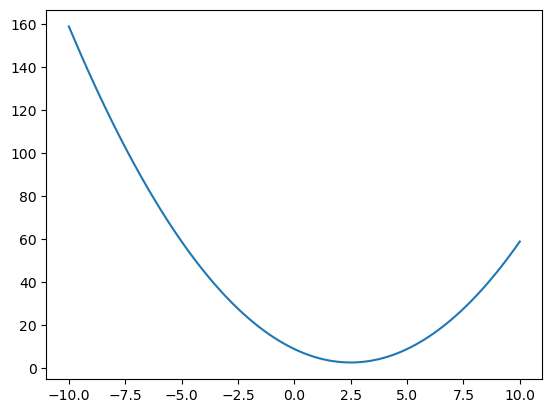You can also plot more than one function on the same graph.

1
2
3
4
ys2 = 10*np.sin(xs) + 20          # ys for the formula y=10sin(x)+20
plt.plot( xs, ys )                # make the original plot
plt.plot( xs, ys2 )               # add the second plot to it
plt.show()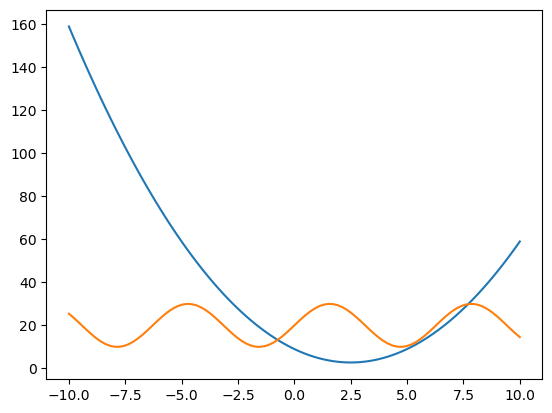See a problem? Tell us or edit the source.

## Using SymPy, in Python

View this solution alone.

This answer assumes you have imported SymPy as follows.

1
2
from sympy import *                   # load all math functions
init_printing( use_latex='mathjax' )  # use pretty math output


You can write a formula and plot it in just a few lines of code.

1
2
3
var( 'x' )
formula = x**2 - 5*x + 9
plot( formula )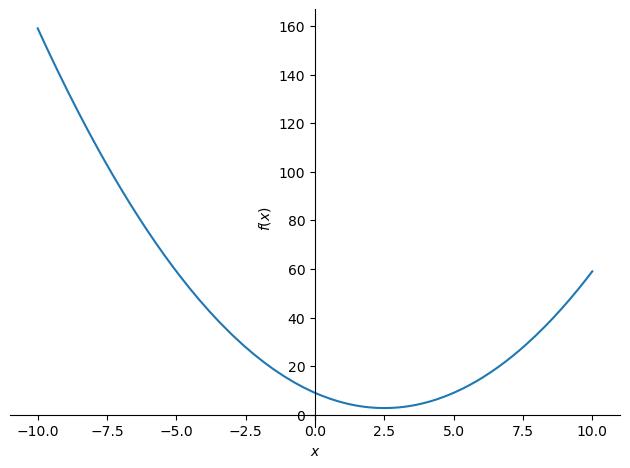1
<sympy.plotting.plot.Plot at 0x7f4b97b97520>


If you want to elimiate the extra bit of text after the graph, just assign the plot to a variable, as in p = plot( formula ).

By default, the graph always covers $x=-10$ to $x=10$. You can change those limits as follows.

1
plot( formula, (x,1,3) )   # just plot from x=1 to x=3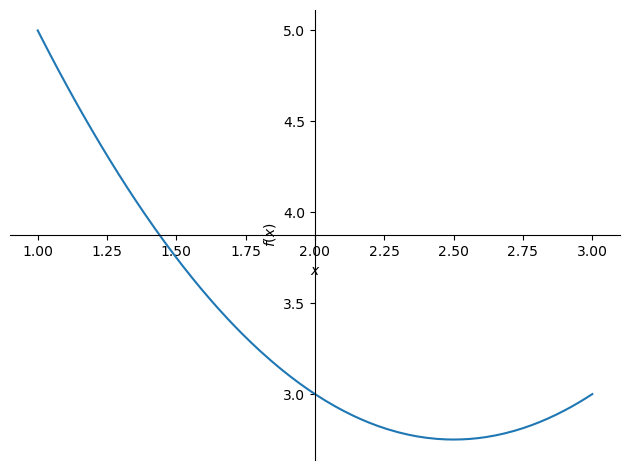1
<sympy.plotting.plot.Plot at 0x7f4bbc097550>


You can also plot more than one function on the same graph.

1
2
formula2 = 10*sin(x) + 20
plot( formula, formula2 )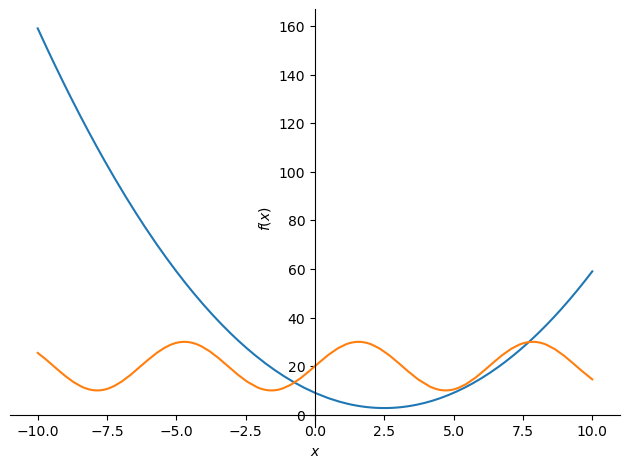1
<sympy.plotting.plot.Plot at 0x7f4b2dcd9c00>


See a problem? Tell us or edit the source.

## Solution, in R

View this solution alone.

Let’s assume we want to graph the function $x^2-5x+9$ from $x=-10$ to $x=10$.

We compute a series of $(x,y)$ pairs to generate the plot. Notice how R automatically computes a $y$ value for each $x$ value if we just include all the $x$s in the formula we wish to graph.

1
2
3
xs <- seq(-10,10,length.out=100)   # 100 values from x=-10 to x=10
ys <- xs^2 - 5*xs + 9              # compute all corresponding ys
plot( xs, ys, type='l' )


You can also plot more than one function on the same graph.

1
2
3
ys2 <- 10*sin(xs) + 20             # ys for the formula y=10sin(x)+20
plot( xs, ys, type='l' )           # make the original plot
lines( xs, ys2 )                   # add the second plot to it